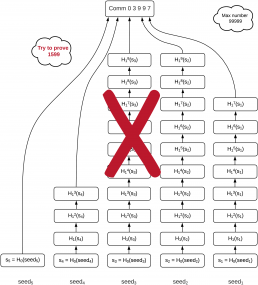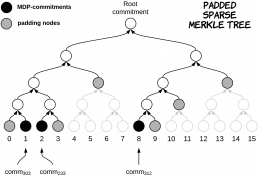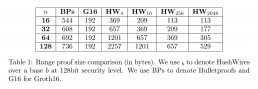– by Kostas Chalkias, Shir Cohen, Kevin Lewi, Fredric Moezinia, and Yolan Romailler.

## Introduction

This post presents one of the easiest-to-implement protocols for proving that a secret integer lies within an interval, without revealing anything else about the number itself. Those familiar with applications of zero-knowledge proofs (ZKP) have probably encountered the concept of range proofs in protocols such as Bulletproofs, MimbleWimble and generic zkSNARK constructions.

But if you ask non-experts to enlist the most important applications of such a mechanism, two common applications mentioned include proof-of-age and proof-of-income to relevant third-parties, such as landlords. Apart from the inconvenience of exposing personal data in these scenarios, some may have privacy and security concerns about how this information is stored once collected.

By looking closer into the requirements, we realized that there exist two substantial differences between applying range proofs for confidential amounts in blockchains and showing your age or income data in real world activities and purchases. Briefly, in the second scenario a) we have a trusted authority that issues credentials and b) typically we don’t perform operations between encrypted values, thus commitment homomorphism is not required. The latter is because we’re interested in proving that some issued credential satisfies a threshold and nothing else. These observations motivated research in the field of credential-based range proofs (CBRP), which differ from regular zero-knowledge range proof solutions in two ways:

• the soundness requirement is relaxed to a weaker notion which we refer to as commitment-conditional soundness (because we already trust issuers, i.e., the identity and passport authorities)
• the zero-knowledge property is relaxed to witness indistinguishability (we just need to hide the exact amount of the issued value)

By taking advantage of these relaxed properties, we describe a new lightweight scheme called HashWires, whose security is based solely upon the security of cryptographically secure hash functions. As we will show, the fact that no advanced math is required (no factorization, no elliptic curves, no pairings, no polynomials) makes this solution surprisingly easy to implement, even in restricted environments, while it compares favorably against Bulletproofs for both 32 and 64 bit numeric values.

To begin with expectations, a HashWires one-time range proof can be just 177 bytes for 32-bit ranges (Vs. 608 bytes in Bulletproofs), while for 64-bit numbers a HashWires proof is 369 bytes (Vs. 692 bytes in Bulletproofs). Performance-wise, carefully selected settings for HashWires allow for 60 times faster proof generation, while verification can be up to 30 times more efficient than a Bulletproofs equivalent range proof.

## Range Proofs from Hashchains

HashWires is inspired by the PayWord protocol, a hash-chain based micro-payments protocol proposed by Rivest and Shamir in 1996. The original idea is very simple and completely based on hash-chain computations.

Briefly, the naive PayWord approach works as follows for our age example: It’s the year 2021 and Alice wants to prove to Carol that she is at least 21 years old without showing her ID or driver license. As every cryptographer might know, Alice was born in 1978 when the RSA paper authors mentioned for the first time “For our scenarios we suppose that A and B (also known as Alice and Bob) are two users of a public-key cryptosystem”. But let’s assume for a while that Carol is not a cryptographer and really needs a proof of over-21 for Alice; also they both trust the government for issued credentials. Additionally, let’s suppose that we want to use this proof system until 2100.

So Alice’s local government (issuer) will provide a signed cryptographic commitment using two collision resistant hash functions $H_0, H_1$ (a single salted hash or HMAC function with different salt/key per $H_0$ and $H_1$ would also work) as follows:

• pick a random $seed$, typically at least 128 bits long
• compute $s = H_{0}(seed)$ and assign this to year 1978 (Alice’s birth year)
• compute $k = 2100 – 1978 = 122$ as the distance from 1978 to the maximum year supported (2100)
• compute the commitment $c = H_{1}^{k}(s)$, which represents $k$ repeated iterations of the function $H_{1}$
• return to Alice $s$ and the signature $sig_c$ over $c$ and Alice’s $photo$.

Thus, Alice is provided with a signed hash-chain commitment and a secret $s$. As we’re in the year 2021, to prove that she was born (at least) 21 years ago, thus at or before 2000, the following protocol is executed:

• Alice computes $d_0 = 2000 – 1978 = 22$
• Alice outputs the proof $p = H_{1}^{d_0}(s)$
• Alice shows $(p, sig_c, photo)$ to Carol
• Carol computes $d_1 = 2100 – 2000 = 100$
• Carol computes the commitment $c = p^{d_1}$, which will hold because $d_0 + d_1 = 122 = k$
• Carol verifies $sig_c$ against the issuer’s known public key (and crosschecks Alice’s photo).

The above works because Carol gets convinced that Alice has some issued secret which is at least 100 hash-chain nodes long, which in turn implies that Alice was born at or before the year 2000 (otherwise the issuer would have never provided Alice with such a long chain). Also, the proof $p$ is literally a single hash value, just 32 bytes as shown below.Despite its simplicity, the above solution suffers from a significant drawback: the cost of commitment, proof generation and eventually proof verification is linear to $k$ in the worst case.

Note that if the granularity of time in the above over-21 example was minutes and not years, we would expect a $k$ at the range of millions, which would inherently require millions of hash invocations. Thus, using PayWord for range proofs is really only suitable for small domains, and its performance for large ranges, i.e., 32 or 64 bit numbers, is not practical.

## Generalizing Hashchains

Before we describe the full solution, let’s consider the case where the issued value is the number 03999 in a system that accepts integers up to 99999. Can we split the single chain somehow, per decimal digit maybe?

Indeed, it would work as follows: The issuer will create 5 chains, one per digit in base10. For the three less significant digits the chain will have a full length of 10 nodes; for the second most important digit the length is 4; and for the most significant digit the length is 1.

Then the issuer will put the top node of each hashchain in an accumulator (i.e., a simple concatenation-then-hash will do the trick of committing to all 5 chains). The values $s_1,..,s_5$ are provided to Alice (prover) who can now also produce the commitment. Obviously, all of $s$ values could be computed via some key derivation function, so that only one seed value would be provided to Alice (that’s her secret).Now, for Alice to prove that she holds a commitment $\geq 1492$, she will provide the yellow (bright) colored nodes as shown in the above figure. Briefly, she starts counting from the top node of each chain and, depending on the digit that she needs to prove, she returns the corresponding node. Thus for 0 1 4 9 2, she will output:

• $s_5$ to represent the first digit (0) – there is no other node for the most significant digit anyway
• ${H_1}^2(s_4)$ to represent the second most significant digit (1)
• ${H_1}^5(s_3)$ for third digit (4) – note that starting from the top, ${H_1}^9(s_3)$ corresponds to number zero, ${H_1}^8(s_3)$ to number one and so on until we reach number four
• $s_2$ for the fourth digit (9)
• ${H_1}^7(s_1)$ for the last digit (2).

Now, after Carol receives the above five values, she will apply as many $H_1$ iterated invocations as the value 0 1 4 9 2 shows. Thus zero times for the first digit, one time for the second digit, four times for the third digit etc. Eventually Carol can compute all the top nodes for each chain and thus be convinced that Alice was issued an integer with a value of at least 01492.

But is this the full story? It seems too easy, right? Let’s try another issued value, 03997 (we just changed the last digit), and now try to prove greater-than-or-equal-to 1599. Hm, but we don’t have a chain of length ten for the last digit. We can prove up to 1597, but not for 1598 and 1599 and in general we cannot prove any number whose last digit is 8 or 9. Unfortunately a single hash multichain cannot work.## Minimum Dominating Partitions

What if we had many hash multichains but somehow ensure that any integer up to 3997 is proof-encodable and at the same time Alice is not able to cheat by proving numbers greater than 3997? Indeed, imagine that the issuer created a separate multi hash-chain commitment for each of the numbers in the following set: $[3997, 3989, 3899, 2999]$.

Then, based on the requested number to prove, Alice would pick one of these commitments: the one that has a long enough hash multichain to encode the number in question. If you understood the logic of hash multichain splitting, then you can easily realize that the commitment to:

• 2999 can prove any number up to 2999
• 3899 can prove any number from 3000 to 3899
• 3989 can prove any number from 3900 to 3989
• 3997 can prove any number from 3990 to 3997

In practice, some commitments can encode other values too, e.g., the commitment to 3989 could be used to prove the number 2350 as well, because its hashchains are long enough for the requirements of 2350. But we can just ignore this property, and always pick the closest greater-than-or-equal commitment to the requested integer, i.e., if we want to prove $\geq 1700$, we would pick the commitment to 2999, but if the requested integer was the number 3950, we would select the commitment to 3989.

It is also obvious that at the same time Alice cannot cheat; she does not own any commitment for a number greater than 3997.

The above numbers in the set are not randomly chosen, they are produced via an algorithm that we call “minimum dominating partition” (MDP), which produces the smallest set-size that satisfies the above properties of being able to prove any range up to the issued value. The algorithm is very efficient and can work for any base (not only base10); here is an example Rust implementation that supports up to 32-bit integers.Some output examples for base10 include [17352, 17349, 17299, 16999, 9999] for 17352, [1399, 999] for 1399 and [8733432, 8733429, 8733399, 8732999, 8729999, 8699999, 7999999] for 8733432. Similarly, [312, 303, 233] is the MDP-list for the number 312 in base4 as shown below:## Reusing Chains

An interesting optimization trick is to share the chains between MDP commitments. Actually this is straightforward via wiring as shown below in the 312 base4 example. Briefly, we create 3 full chains, one per digit. Then, each MDP commitment is wired to its corresponding indices as shown below:This wiring technique was the inspiration for naming this protocol “HashWires”, which allows for significant optimizations on proof generation, especially for large domains that would typically require dozens of MDP commitments.

## Hiding the MDP Population

It’s important to highlight that the size of the MDP-list can go up to the maximum number of digits supported in each application. For instance, if the maximum acceptable number consists of 20 decimal digits, the size of MDP-list can reach up to 20 elements in base10. Similarly, for numbers up to to $2^{64}-1$ using base16 we would expect a maximum of 16 MDP values in the worst case.

However, the size of the MDP-list and potentially the index of the selected MDP commitment might leak information about the issued number. Why? Imagine that the number 02999 was issued (out of the maximum possible 99999). This number however requires only one element in the MDP-list, the , as it can be used to prove any number $\leq 2999$. If Alice however revealed this information (that there exists only one MDP commitment), a verifier learns that the issued number cannot be 2998 or any other integer that would require more than one MDP values.

Thus, we always need to pad the MDP-list (+ shuffle it), so verifiers have no information about the MDP-list size when they see a HashWires proof.

Although an RSA accumulator could work, that would not be post-quantum secure and the idea is to avoid factorization or elliptic curves anyway. Similarly, a plain Merkle tree where we add random leaves would be an option, but the padded sparse Merkle tree accumulator is recommended to reduce the number of padding nodes required and still rely on hash functions only. A Rust implementation of the padded sparse tree is available in the smtree crate (library).## The Final HashWires Protocol

There are more optimization tricks and extensions that we could apply on top of the described protocol, for example related to how we can truncate the prefix zero digits of small issued values and add malleability protection. One can learn more from the “HashWires: Hyperefficient Credential-Based Range Proofs” paper available on ePrint. An interesting analogy is to see HashWires as an extended variant of Winternitz one-time post-quantum signatures (WOTS). As in WOTS, the basic HashWires protocol is an one-time range proof system and can be extended to stateful many-times using a Merkle tree of one time proofs. Making HashWires many-time stateless is still an open research problem. Moreover, the bigger the selected base, the longer the hashchains, but the shorter the proof. Typically, base16 or base256 are considered practical for today’s requirements of usually proving ranges to values up to 64 bits that work for most real world applications.

Applications of HashWires include range proofs for KYC credentials, location (you can easily combine two proofs, one per coordinate, to create a range in the 2D or 3D space), timestamp ranges (i.e., in digital certificates we’re only interested in cert validation, but not necessarily when it was issued) and finally micro-payments, auctions and gradually redeemable bank checks.As for the concrete results, we provide some comparison tables taken by comparing Hashwires for different bases against Bulletproofs and Groth16.